# WebAssembly - JavaScript API

In this chapter, we will understand how to load the wasm code and execute them in the browser using the help of javascript webassembly API.

Here are some important API's, we are going to make use throughout the tutorial to execute wasm code.

• fetch() Browser API
• WebAssembly.compile
• WebAssembly.instance
• WebAssembly.instantiate
• WebAssembly.instantiateStreaming

Before we discuss the WebAssembly javascript API's, to test the API and the output we are going to use the following C program and the .wasm code generated from the c program using wasm explorer.

An example for C Program is as follows −

```#include<stdio.h>
int square(int n) {
return n*n;
}
```

We will make use of WASM explorer, to get the wasm code −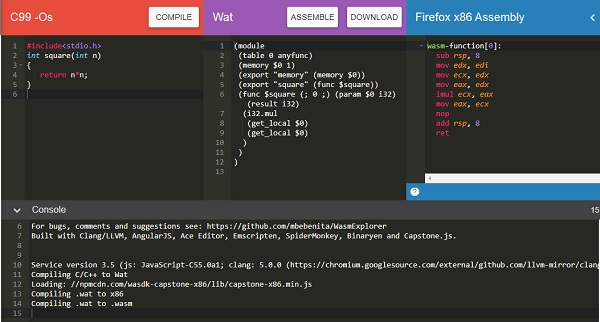## fetch() Browser API

fetch() API is meant to load .wasm network resource.

```<script>
var result = fetch("findsquare.wasm");
console.log(result);
</script>
```

It returns a promise as shown below −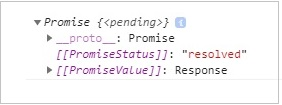You can also make use of XMLHttpRequest method to fetch the wasm network resource.

## WebAssembly.compile()

The api responsibility is to compile the module details that are fetched from .wasm.

### Syntax

The syntax is as given below −

```WebAssembly.compile(buffer);
```

### Parameters

Buffer − This code from .wasm has to be converted to a typed array or arraybuffer, before giving as input to compile.

### Return value

It will return a promise that will have the compiled module.

### Example

Let us see one example, that gives the output as a compiled module using webAssembly.compile().

```<script>
fetch("findsquare.wasm") .then(bytes => bytes.arrayBuffer())
.then(mod => {
var compiledmod = WebAssembly.compile(mod);
compiledmod.then(test=> {
console.log(test);
})
})
</script>
```

### Output

The console.log, when checked in the browser, will give you the compiled module details −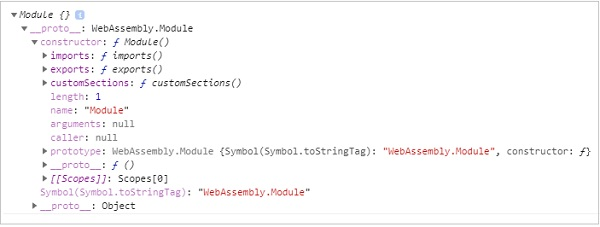The module has a constructor object with imports, exports, and customSections. Let us see the next API, to get more details of the compiled module.

## WebAssembly.instance

Using the WebAssembly.instance, API will give you the executable instance of the compiled module that can be further executed to get the output.

### Syntax

The syntax is as given below −

```new WebAssembly.Instance(compiled module)
```

### Return value

The return value will be an object with the array of exports function that can be executed.

### Example

```<script>
fetch("findsquare.wasm")
.then(bytes => bytes.arrayBuffer())
.then(mod => WebAssembly.compile(mod)).then(module => {
let instance = new WebAssembly.Instance(module);
console.log(instance);
})
</script>
```

### Output

The output will give us an array of exports function as shown below −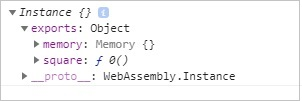You can see the square function, that we got from the C code that is compiled.

To execute the square function, you can do the following −

```<script>
fetch("findsquare.wasm")
.then(bytes => bytes.arrayBuffer())
.then(mod => WebAssembly.compile(mod))
.then(module => {
let instance = new WebAssembly.Instance(module);
console.log(instance.exports.square(15));
})
</script>
```

The output will be −

```225
```

## WebAssembly.instantiate

This API takes care of compiling and instantiating the module together.

### Syntax

The syntax is as follows −

```WebAssembly.instantiate(arraybuffer, importObject)
```

### Parameters

arraybuffer − The code from .wasm has to be converted to typed array or arraybuffer before giving as input to instantiate.

importObject − The import object has to have details of the memory, imported functions to be used inside the module. It can be an empty module object, in case, there is nothing to be shared.

### Return value

It will return a promise, that will have module and instance details.

### Example

```<script type="text/javascript">
const importObj = {
module: {}
};
fetch("findsquare.wasm")
.then(bytes => bytes.arrayBuffer())
.then(module => WebAssembly.instantiate(module, importObj))
.then(finalcode => {
console.log(finalcode); console.log(finalcode.instance.exports.square(25));
});
</script>
```

### Output

When you execute the code, you will get the below mentioned output.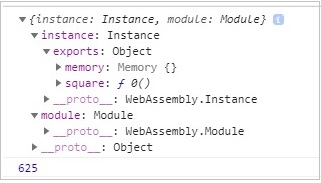## WebAssembly.instantiateStreaming

This API takes care of compiling as well as instantiating the WebAssembly module from the .wasm code given.

### Syntax

The syntax is as given below −

```WebAssembly.instantiateStreaming(wasmcode, importObject);
```

### Parameters

wasmcode − Response from fetch or any other API that gives the wasm code and returns a promise.

importObject − The import object has to have details of the memory, imported functions to be used inside the module. It can be an empty module object in case there is nothing to be shared.

### Return Value

It will return a promise, that will have module and instance details.

### Example

An example is discussed below −

```<script type="text/javascript">
const importObj = {
module: {}
};
WebAssembly.instantiateStreaming(fetch("findsquare.wasm"), importObj).then(obj => {
console.log(obj);
});
</script>
```

When you test it in the browser, you will see an error −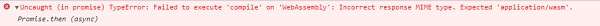To make it work at your server end, you will have to add the mime type application/wasm or else make use of WebAssembly.instantiate(arraybuffer, importObject).